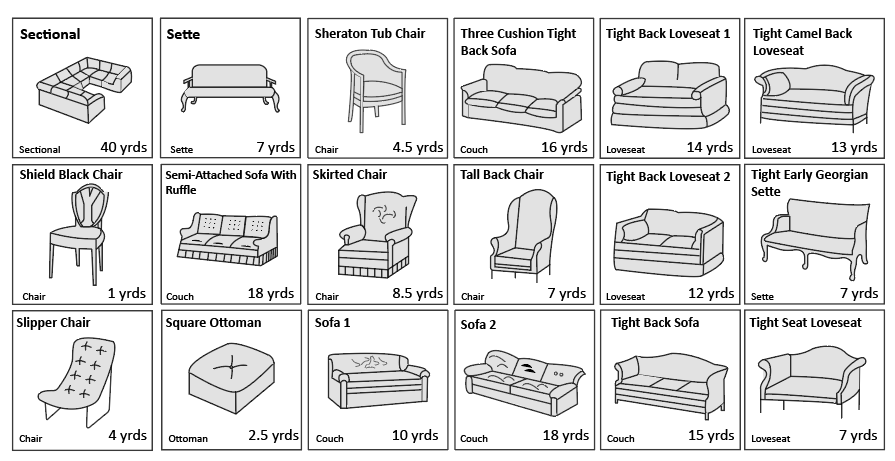Uh Oh! It seems you’re using an Ad blocker!

We always struggled to serve you with the best online calculations, thus, there's a humble request to either disable the AD blocker or go with premium plans to use the AD-Free version for calculators.

Or# Fabric Calculator

Quickly calculate the quantity of the fabric required to complete a particular project by giving a couple of simple inputs to this fabric estimator.

Fabric Width:

Pieces To Cut Width:

Pieces To Cut Length:

Number Of Pieces:

unit:Table of Content

Get the Widget!

Add this calculator to your site and lets users to perform easy calculations.

Feedback

How easy was it to use our calculator? Did you face any problem, tell us!

Utilize the sailrite fabric calculator to determine how much fabric you should buy for a project based on the number of pieces that you need to cut.

A precise calculation will let you have the correct quantity of fabric for your project.

## How to Calculate The Amount of Fabric You’ll Need For Your Project?

Go through the following steps to calculate the amount of fabric required for a specific project:

• First of all, determine the number of pieces that you can get from the width of the fabric by dividing the fabric width by the width of one piece. Round down the result.

Number of Pieces = fabric width/width of each piece

• Now in this step calculate the number of rows by dividing the total number of pieces that you need by the number of pieces that are in your width.

Number of Rows = total number of pieces/number of pieces in width

• Calculate the total length by multiplying the number of rows with the length of each piece and you will have the exact measurement of the fabric.

Total Length = number of rows x length of each piece

• Divide the result by 36 inches to convert it into yards.

If it seems difficult then opt for a good sailrite calculator as it will let you perform the precise calculation effortlessly.

### Practical Example:

Let’s suppose you have a 56-inch-wide fabric and need pieces that are 7 inches square. And you need 24 7-inch squares. Now how do calculate the fabric?

### Solution:

Given That:

Fabric Width = 56 inch

Pieces Size = 7 inches square

Number of Pieces = fabric width/width of each piece

Number of pieces = 56 / 7 = 8

Number of rows = total number of pieces/number of pieces in width

Number of rows = 24 / 8 = 3

Total Length = number of rows x length of each piece

Total length of material required = 3 x 7 = 21

Rather than dragging yourself into this complex calculation, simply get the help of a free fabric yardage calculator and perform the whole calculation in seconds without any chance of mistakes.

## Furniture Upholstery Yardage Chart:

The following chart is very useful in calculating the correct fabric for your furniture items.## Common Cuts of Yardage:

In the following table, we have provided the common fabric cuts in yards and their corresponding values in inches.

 YARDAGE INCHES 1/4 9 1/3 12 1/2 18 2/3 24 3/4 27 1 36 2 72 3 108

## How Does Our Free Fabric Calculator Work?

Estimate the exact fabric needed for a project with the help of our handy fabric yard calculator.

### What Do You Need To Enter?

It only requires a couple of inputs such as:

• Fabric width
• Piece width
• Length
• The number of pieces required
• Unit

### This Is What You Will Get!

• It provides you with the quantity of the material required for a project.
• Number of pieces
• Instant and accurate results.

This yardage calculator is a very useful tool for home improvement projects where you need multiple pieces of the same size of an item according to a standard measurement.

## FAQ’s:

### How Many Square Feet Are In A Yard of Fabric?

One yard of fabric is equal to 18 square feet of fabric.

### How Do You Calculate Fabric For Furniture?

For this, measure the back, front, and sides and add one inch for seam allowance. Now measure the frame that you have to cover with the fabric. After that divide each measurement to calculate the yardage or simply get the help of a yards of fabric calculator to perform the swift calculations.

### What Does 1 Yard of Fabric Look Like?

When you are dealing with fabric, one yard is equal to 36”, 3 feet, or 0.9144 meters and 91.44 cm. Meanwhile, it equals to the double of a shoulder width.

## References:

From the source of marthastewart.com: How to calculate the amount of fabric you’ll need?